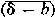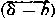# Calculus of Observations

The following article is from The Great Soviet Encyclopedia (1979). It might be outdated or ideologically biased.

## Calculus of Observations

the application of mathematical methods to results of observations for constructing conclusions about the true values of unknown quantities.

Every result of observations related to measurements involves errors of different origin. Errors are divided into three groups in terms of their nature: gross, systematic, and random (we will henceforth assume that observations do not contain gross errors). The measurement result Y of some quantity μ is usually assumed to be a random variable; therefore, the measurement error δ = Y —μ will also usually be a random variable. Suppose b = Eδ is the mathematical expectation of the error. Then Y = μ + b + (δ —b). The value b is said to be the systematic error, and δ —b is the random error; the mathematical expectation of δ —b is equal to zero.

The systematic error b is often known in advance and thus can be easily eliminated. In astronomy, for example, in measuring the size of the angle between the direction to a star and the plane of the horizon, the systematic error is the sum of two errors: the systematic error caused by the instrument used in the process of reading the given angle and the systematic error resulting from the refraction of light rays in the atmosphere. The instrument error is determined by means of an error table or graph for the given instrument; the error caused by refraction (for zenith angles less than 80°) can be calculated from theory to sufficient accuracy.

The influence of random errors is estimated using methods of the theory of errors. If Y1, Y2, . . . , Yn are the results of n independent measurements of μ conducted under identical conditions and using the same means, then we usually take

(1)μ ~; Ȳ − b = [(Y1 + ... + Yn)/n] − b

where b is the systematic error. In order to estimate the absolute error of the approximation (1), see articles , LEAST SQUARES, METHOD OF and SIGNIFICANCE LEVEL OF A STATISTICAL TEST.

Whenever it is required to calculate the value of some function f(y) at the point y = μ, the quantity μ being estimated in terms of η independent observations Y1, Y2, . . . , Yn, we generally assume

(2)f (μ) Δ; f (̄b)

Suppose B is the mathematical expectation of the quantity

(3)Δ = f (b) —f (μ)

that is,

f (b) = f (μ) + B + (Δ − B)

Then B is the systematic error and (Δ —B) is the random error of the approximate equation (2). If the random errors of the independent observations Y1, Y2, . . . ,Yn obey the same distribution and if the function f(y) differs little from a linear function in the neighborhood of the point y = μ, then B Δ; 0 and, whereis the arithmetic mean of the random errors of the initial observations. That is, if E(δi - b)2 = σ2, for i = 1, 2, . . . , n. then E(Δ - B) 2Δ; EΔ2 ~; [f(μ)]1/2σ2/n/η 0 as η ∞.

The calculus of observations often makes use of the method of least squares for the case of several unknown parameters.

If a dependence between the random variables X and Y is studied on the basis of a set of η independent observations, each of which is a vector (Xi,Yi), i = 1, . . . , n, whose components Xi; and yi obey a given joint distribution for the variables X and Y, then the corresponding calculus of observations makes use of correlation theory and mathematical statistics.

Certain assumptions and hypotheses must be made in the calculus of observations regarding the nature of a functional dependence, the distribution of random errors, and so on. Therefore, the calculus of observations must include a test for the agreement between the assumptions made and the results of observations used.

### REFERENCES

Whittaker, E. T. , and G. Robinson. Matematicheskaia obrabotka rezul’tatov nabiiudenii. Leningrad-Moscow, 1935. (Translated from English.)
Linnik, Iu. V. Metod naimen ‘shikh kvadratov i osnovy matematiko-statisticheskoi teorii obrabotk i nabliudenii, 2nd ed. Moscow, 1962.

L. N. BOL’SHEV